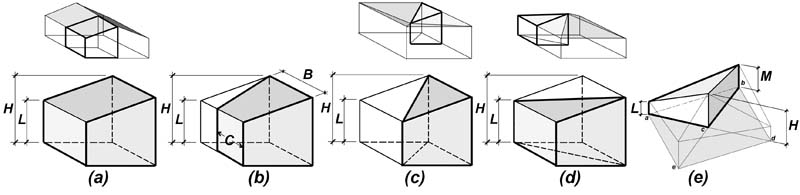Building technology calculators
contact

# Efficiency of tapered roof insulation calculator

Jonathan Ochshorn

Introduction: The R-value of tapered roof insulation is often found by using the average thickness of the insulation to determine an "average" R-value. However, the true average R-value differs from this assumed value, sometimes significantly. To determine the actual R-value of tapered insulation, multiply the assumed R-value (based on either the arithmetic mean average thickness or the true average thickness) by the "efficiency" value provided by this calculator.

Alternatively, use this interactive online example to calculate the true heat loss through a roof with tapered insulation considering the efficiency of the tapered insulation.

A detailed explanation of tapered insulation and R-Values can be found in my paper: Determining the Average R-Value of Tapered Insulation

Directions:

For each geometric component of the roof (e.g., 1-way tapers, 4-way tapers, crickets, etc.), enter values for high- and low-point thickness (as well as intermediate thickness for crickets only), and ratio of sides B : C for trapezoidal solids only (see Figure 1-b). These various parameters and geometries are illustrated in Figure 1. One cannot separate the R-value calculation of tapered insulation from the R-value calculation of other roof elements such as ceiling finishes or structural slabs: the entire assembly must be evaluated as a unit. For that reason, it is important to include the assumed-constant R-value of all additional roof materials, and the R-value per unit thickness for the tapered insulation.

In addition, enter "yes" or "no" for the consideration of curved heat-flow trajectories ("taper slope"). Commonly-used taper slopes are flat enough so that the effect of curved heat flow trajectories can be neglected, i.e., for slopes of 1 in. per foot (1:12) or less.

Press "update" button.Fig. 1. For purposes of calculation, the basic tapered insulation forms are as follows: (a) 1-way slope or 2-way slope; (b) trapezoidal slope with parallel high- and low-point sides; (c) 1-way slope converging down to a point, or 4-way slope with interior drainage; (d) 1-way slope converging up to a point, or 4-way slope with exterior drainage; and (e) triangular cricket

The calculated efficiencies, expressed as percentages, are based on an assumed "average" insulation thickness of (H + L)/2, where H and L are the high- and low-point thicknesses (or R-values) of the tapered insulation; or on the true average thickness (R-value) of the tapered insulation, computed by dividing the volume of material by its area.